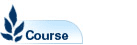# Mathematics 2A - MATH2069

Faculty: Faculty of Science

Course Outline: http://www.maths.unsw.edu.au

Campus: Sydney

Units of Credit: 6

Indicative Contact Hours per Week: 6

Enrolment Requirements:

Prerequisite: MATH1231 or MATH1241 or MATH1251; Exclusions: MATH2011, MATH2111, MATH2510, MATH2610, MATH2520 & MATH2620

Tuition Fee: See Tuition Fee Schedule

Further Information: See Class Timetable

View course information for previous years.

## Description

Several Variable Calculus:
Vectors, differential calculus of curves in R3 and surfaces, Taylor series for functions of two variables, critical points, local maxima and minima. Lagrange multipliers, integral calculus for functions of several variables using various co-ordinate systems, conservative vector fields and line integrals, Green's Theorem in the plane, divergence and curl, surface integrals, Stokes' Theorem, Gauss' divergence Theorem.

Complex Analysis:
Complex numbers, simple mapping problems, differentiation theory for complex functions, Cauchy Riemann equations, analytic functions, the elementary functions, Integration Theory for complex functions, Cauchy's Theorem and the Cauchy integral formulae, Taylor series and Laurent Series, residues, evaluating real integrals and trigonometric integrals using residues.

Note: Available only to students for whom it is specifically required as part of their program